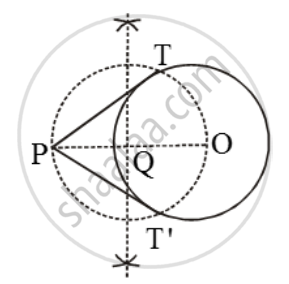# Draw a circle of radius 3 cm. Take a point at a distance of 5.5 cm from the centre of the circle. From point P, draw two tangents to the circle. - Mathematics

Sum

Draw a circle of radius 3 cm. Take a point at a distance of 5.5 cm from the centre of the circle. From point P, draw two tangents to the circle.

#### Solution

Steps of ConstructionStep I: Take a point O in the plane of the paper and draw a circle of radius 3 cm.

Step II: Mark a point P at a distance of 5.5 cm from the centre O and join OP.

Step III: Draw the right bisector of OP, intersecting OP at Q.

Step IV: Taking Q as centre and OQ = PQ as radius, draw a circle to intersect the given circle at T and T´.

Step V : Join PT and PT´ to get the required tangents.

Concept: Construction of Tangents to a Circle
Is there an error in this question or solution?
Share